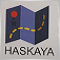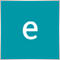# How to avoid order modify error1?395

Hi, I have already some orders with tp and sl.

But I want to scan all orders and modify ONLY tp=0.

Here is my code.

``` void TP_zero(){
for (int aa= OrdersTotal() - 1; aa>= 0; aa--) {
if (OrderSelect(aa, SELECT_BY_POS, MODE_TRADES)){

OrderModify(OrderTicket(), OrderOpenPrice(),OrderStopLoss() 0, White);

}
}}```

But there is some issues.

Order has already stoploss and takeprofit value. I want to change only takeprofit=0.

I don't want to change stoploss, so I used OrderStopLoss() and modify tp=0.

But with this code , I have Order modify error1 because OrderStoploss is modify with the same price.

How can I solve this?2429

Cromo:

Hi, I have already some orders with tp and sl.

But I want to scan all orders and modify ONLY tp=0.

Here is my code.

But there is some issues.

Order has already stoploss and takeprofit value. I want to change only takeprofit=0.

I don't want to change stoploss, so I used OrderStopLoss() and modify tp=0.

But with this code , I have Order modify error1 because OrderStoploss is modify with the same price.

How can I solve this?

```//+------------------------------------------------------------------+
//|                                                          ttt.mq4 |
//|                                        Copyright 2021, HaskayaFx |
//|                                   https://www.haskayayazilim.net |
//+------------------------------------------------------------------+
#property copyright "Copyright 2021, HaskayaFx"
#property link      "https://www.haskayayazilim.net"
#property version   "1.00"
#property strict
input double StopLost=300;
input double TakeProfit=600;
//+------------------------------------------------------------------+
//| Expert initialization function                                   |
//+------------------------------------------------------------------+
int OnInit()
{
//---

//---
return(INIT_SUCCEEDED);
}
//+------------------------------------------------------------------+
//| Expert deinitialization function                                 |
//+------------------------------------------------------------------+
void OnDeinit(const int reason)
{
//---

}
//+------------------------------------------------------------------+
//| Expert tick function                                             |
//+------------------------------------------------------------------+
void OnTick()
{
//---

}

int TP_zero()
{

double SL,TP;
bool ticket;

for(int i = 0; i < OrdersTotal(); i++)
{

if(OrderSelect(i,SELECT_BY_POS,MODE_TRADES)==false)   break;
string OrderMagic=string(OrderMagicNumber());

// TP=TakeProfit*MarketInfo(OrderSymbol(),MODE_BID);
// SL=StopLost*MarketInfo(OrderSymbol(),MODE_ASK);
TP=TakeProfit*MarketInfo(OrderSymbol(),MODE_DIGITS);
SL=StopLost*MarketInfo(OrderSymbol(),MODE_DIGITS);

if((OrderType()==OP_BUY || OrderType()==OP_SELL) &&( OrderStopLoss()!=SL ||(OrderStopLoss()==0)))
ticket= OrderModify(OrderTicket(), OrderOpenPrice(),
NormalizeDouble(SL,int(MarketInfo(OrderSymbol(),MODE_DIGITS))),
NormalizeDouble(TP,int(MarketInfo(OrderSymbol(),MODE_DIGITS))),
0,
CLR_NONE);

}

return(0);
}

//+------------------------------------------------------------------+
```89

Cromo:

Hi, I have already some orders with tp and sl.

But I want to scan all orders and modify ONLY tp=0.

Here is my code.

But there is some issues.

Order has already stoploss and takeprofit value. I want to change only takeprofit=0.

I don't want to change stoploss, so I used OrderStopLoss() and modify tp=0.

But with this code , I have Order modify error1 because OrderStoploss is modify with the same price.

How can I solve this?

try this

``` void TP_zero()
{
for (int aa= OrdersTotal() - 1; aa>= 0; aa--)
{
OrderSelect(aa, SELECT_BY_POS, MODE_TRADES)

OrderModify(OrderTicket(), OrderOpenPrice(),OrderStopLoss(),
0 ,0, clrwhite);

}
}```Moderator
29903

Always use
#property strict
in your codes.
When starting a project use the "New" button top left of the editor. #property strict will be included automatically.

If the compiler issues a warning don't ignore it, fix it.

Following examples not tested.

```   OrderSelect(0, SELECT_BY_POS, MODE_TRADES);
//will give you the error that the return value of OrderSelect should be checked. This is simple to do
if(OrderSelect(0, SELECT_BY_POS, MODE_TRADES))
{
//code to deal with the selected order
}
OrderModify(OrderTicket(), OrderOpenPrice(),OrderStopLoss(),0,0, clrWhite);
//will give you the error that the return value of OrderModify should be checked. The minimum that should be done is to print the error if it fails
if(!OrderModify(OrderTicket(), OrderOpenPrice(),OrderStopLoss(),0,0, clrWhite))
{
Print("Modifying Ticket #",OrderTicket()," failed with error code ",GetLastError());
}
OrderSend(_Symbol,OP_BUY,0.1,Ask,20,0,0,NULL,12345,0,clrNONE);
//will give you the error that the return value of OrderModify should be checked. The minimum that should be done is to print the error if it fails
int ticket=OrderSend(_Symbol,OP_BUY,0.1,Ask,20,0,0,NULL,12345,0,clrNONE);
if(ticket==-1)
{
Print("OrderSend for Buy order failed with error code ",GetLastError());
}
//You will often see people silence the compiler by using
bool result=OrderModify(OrderTicket(), OrderOpenPrice(),OrderStopLoss(),0,0, clrWhite);
int ticket=OrderSend(_Symbol,OP_BUY,0.1,Ask,20,0,0,NULL,12345,0,clrNONE); //No print if an error occurs!
//Sure, you don't get the compiler warnings, but it won't help you to track down any errors!

//A very common error when modifying an order is to try to modify the SL or TP with the same as the existing SL or TP (error 1)
for(int x=OrdersTotal()-1; x>=0; x--)
{
if(OrderSelect(x,SELECT_BY_POS) && OrderSymbol()==_Symbol && OrderMagicNumber()==MagicNumber)
{
double nsl=0;
if(OrderType()==OP_BUY)
{
nsl=Low;
}
else if(OrderType()==OP_SELL)
{
nsl=High;
}
else continue;
if(MathAbs(OrderStopLoss()-nsl)>Point/2)
if(!OrderModify(OrderTicket(), OrderOpenPrice(),nsl,OrderTakeProfit(),0, clrWhite))
{
Print("Modifying Ticket #",OrderTicket()," failed with error code ",GetLastError(),
" Old SL ",DoubleToString(OrderStopLoss(),Digits)," New SL ",DoubleToString(nsl,Digits));
}
}
}```Moderator
29903

When posting code always make sure that it compiles.

Cromo:

``` void TP_zero(){
for (int aa= OrdersTotal() - 1; aa>= 0; aa--) {
if (OrderSelect(aa, SELECT_BY_POS, MODE_TRADES)){

OrderModify(OrderTicket(), OrderOpenPrice(),OrderStopLoss() 0, White);

}
}}```

You are missing the , after OrderStopLoss()

ejmin ejoni:

``` void TP_zero()
{
for (int aa= OrdersTotal() - 1; aa>= 0; aa--)
{
OrderSelect(aa, SELECT_BY_POS, MODE_TRADES)

OrderModify(OrderTicket(), OrderOpenPrice(),OrderStopLoss(),
0 ,0, clrwhite);

}
}```

No such colour as clrwhite, it is clrWhite.Moderator
29903

Mehmet Bastem:
```//+------------------------------------------------------------------+
//|                                                          ttt.mq4 |
//|                                        Copyright 2021, HaskayaFx |
//|                                   https://www.haskayayazilim.net |
//+------------------------------------------------------------------+
#property copyright "Copyright 2021, HaskayaFx"
#property link      "https://www.haskayayazilim.net"
#property version   "1.00"
#property strict
input double StopLost=300;
input double TakeProfit=600;
//+------------------------------------------------------------------+
int TP_zero()
{

double SL,TP;
bool ticket;

for(int i = 0; i < OrdersTotal(); i++)
{

if(OrderSelect(i,SELECT_BY_POS,MODE_TRADES)==false)   break;        //Why break if the select fails?
string OrderMagic=string(OrderMagicNumber());                       //Why get this if you don't use it?
//No check of the order's symbol or magic number

TP=TakeProfit*MarketInfo(OrderSymbol(),MODE_BID);                //TP = Bid*600????
SL=StopLost*MarketInfo(OrderSymbol(),MODE_ASK);                  //SL = Ask*300????
//You can only use MarketInfo if the symbol is the chart symbol

if((OrderType()==OP_BUY || OrderType()==OP_SELL) &&( OrderStopLoss()!=SL ||(OrderStopLoss()==0))) //Be careful comparing doubles
ticket= OrderModify(OrderTicket(), OrderOpenPrice(),              //Note in my earlier post about just silencing the compiler
NormalizeDouble(SL,int(MarketInfo(OrderSymbol(),MODE_DIGITS))),  //You can only use MarketInfo if the symbol is the chart symbol
NormalizeDouble(TP,int(MarketInfo(OrderSymbol(),MODE_DIGITS))),  //You can only use MarketInfo if the symbol is the chart symbol
0,
CLR_NONE);

}

return(0);  //function may as well be void as it always will return 0
}

//+------------------------------------------------------------------+```2429

Keith Watford:

the code below can afford

```// TP=TakeProfit*MarketInfo(OrderSymbol(),MODE_BID);
// SL=StopLost*MarketInfo(OrderSymbol(),MODE_ASK);
TP=TakeProfit*MarketInfo(OrderSymbol(),MODE_DIGITS);
SL=StopLost*MarketInfo(OrderSymbol(),MODE_DIGITS);```

this part has been overlooked.

The code below is to set all open

```         if((OrderType()==OP_BUY || OrderType()==OP_SELL) &&( OrderStopLoss()!=SL ||(OrderStopLoss()==0)))
ticket= OrderModify(OrderTicket(), OrderOpenPrice(),
NormalizeDouble(SL,int(MarketInfo(OrderSymbol(),MODE_DIGITS))),
NormalizeDouble(TP,int(MarketInfo(OrderSymbol(),MODE_DIGITS))),
0,
CLR_NONE);```

orders SL and TP on any pair. So not just for 1 parity.

It is not important . Because SL and TPs will be updated until you get here.

` return(0);  //function may as well be void as it always will return 0`Moderator
29903

Mehmet Bastem:

I see that you have edited your code

```input double StopLost=300;
input double TakeProfit=600;```
```         TP=TakeProfit*MarketInfo(OrderSymbol(),MODE_DIGITS);
SL=StopLost*MarketInfo(OrderSymbol(),MODE_DIGITS);```

The SL and TP still don't make any sense. You must think about your calculations.

Say the order was for EURUSD and the current price is 1.20000

Digits is 5

TP=600*5 which is 3000

SL=300*5 which is 1500

Do those values seem acceptable for a symbol with a price of 1.20000 ?2429

Keith Watford:

I see that you have edited your code

The SL and TP still don't make any sense. You must think about your calculations.

Say the order was for EURUSD and the current price is 1.20000

Digits is 5

TP=600*5 which is 3000

SL=300*5 which is 1500

Do those values seem acceptable for a symbol with a price of 1.20000 ?

If you look at the first code I wrote, it has been corrected. When I was going to write Digists by mistake, ASK or BID was written.

``` // TP=TakeProfit*MarketInfo(OrderSymbol(),MODE_BID);
// SL=StopLost*MarketInfo(OrderSymbol(),MODE_ASK);
TP=TakeProfit*MarketInfo(OrderSymbol(),MODE_DIGITS);
SL=StopLost*MarketInfo(OrderSymbol(),MODE_DIGITS);```395

Mehmet Bastem:

If you look at the first code I wrote, it has been corrected. When I was going to write Digists by mistake, ASK or BID was written.

thank you so many advice.

I am kind of confused with many code.....
Let me check one by one.